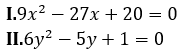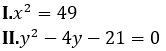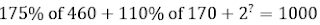Dear Aspirants,

Quantitative Aptitude Quiz For SBI PO/Clerk Prelims

Numerical Ability or Quantitative Aptitude Section has given heebie-jeebies to the aspirants when they appear for a banking examination. As the level of every other section is only getting complex and convoluted, there is no doubt that this section, too, makes your blood run cold. The questions asked in this section are calculative and very time-consuming. But once dealt with proper strategy, speed, and accuracy, this section can get you the maximum marks in the examination. Following is the Quantitative Aptitude quiz to help you practice with the best of latest pattern questions.

Directions (1-5): Study the following bar graph to answer the questions that follow.
Total vacancy in three different departments of central government
Agriculture = 1,50,000
IT department = 2,45,000
Home Ministry = 2,75,000Q1. The employees working in Agriculture and IT departments together in year 2007 are approximately what percent of employees working in Home Ministry in the same year ?
78%
74%
82%
84%
95%

Q2. Find the approximate average number of employees working in IT department over all years.
95,500
90,000
88,812
10,550
65,000

Q3. In which department, total number of vacant seats is the most among all the three departments ?
Agriculture
Home ministry
Can’t determined
IT
None of these

Q4. Find the ratio of employees working in Agriculture and IT departments together in year 2008 to the employees working in Home ministry in the same year.
73 : 66
66 :73
55 : 76
83 : 65
65 : 83

Q5. What is the difference between average number of employees working in IT department over all years and average number of employees working in home ministry over all years ?
50,000
45,250
48,500
55,500
35,500

Directions (6-10) : In each of these questions, two equations numbered I and II are given. You have to solve both the equation and give answer

Q6.if x < y
if x ≤ y
if x > y
if x ≥ y
if x = y or the relationship cannot be established

Q7.if x < y
if x ≤ y
if x > y
if x ≥ y
if x = y or the relationship cannot be established

Q8.if x < y
if x ≤ y
if x > y
if x ≥ y
if x = y or the relationship cannot be established

Q9.if x < y
if x ≤ y
if x > y
if x ≥ y
if x = y or the relationship cannot be established

Q10.if x < y
if x ≤ y
if x > y
if x ≥ y
if x = y or the relationship cannot be established

Directions (11-15): What value should come in place of question mark (?) in the following questions?

Q11.Q12.1170
1710
1510
1700
1960

Q13.3
4
5
2
1

Q14. 80% of 650 – 25 % of ? = 60% of 440
1210
1024
1035
1004
1002

Q15. 3.2% of 500 × 2. 4% of ? = 288
650
700
600
750
850

You May also like to Read: